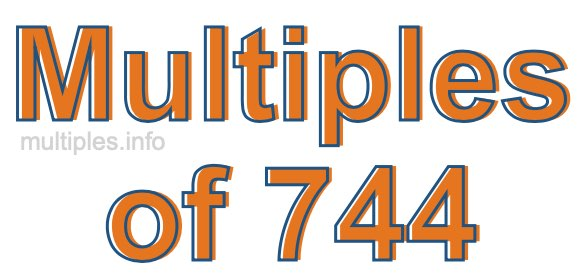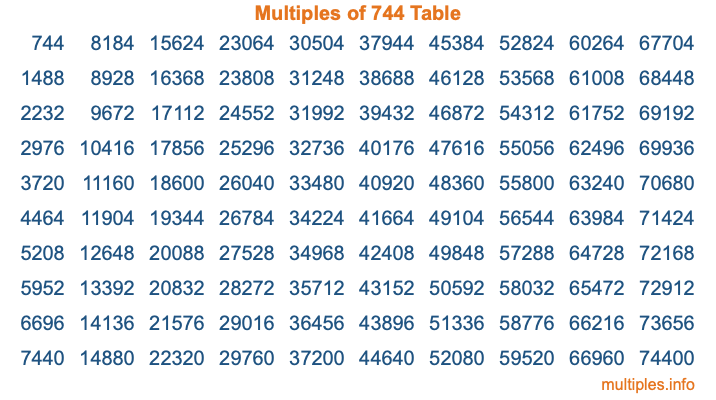Multiples of 744Welcome to the Multiples of 744 page. Here we will first teach you everything you will ever need to know about the multiples of 744, and then give you a study guide summary of everything we taught you to make sure you remember it all. Use this page to look up facts and learn information about the multiples of 744. This page will make you a multiples of seven hundred forty-four expert!

Definition of Multiples of 744
Multiples of 744 are all the numbers that when divided by 744 equal an integer. Each of the multiples of 744 are called a multiple. A multiple of 744 is created by multiplying 744 by an integer.

Therefore, to create a list of multiples of 744, you start with 1 multiplied by 744, then 2 multiplied by 744, then 3 multiplied by 744, and so on for as long as you want. Thus, the list of the first five multiples of 744 is 744, 1488, 2232, 2976, and 3720. To see a larger list of multiples of 744, see the printable image of Multiples of 744 further down on this page. We also have a category where you can choose any nth multiple of 744.

Multiples of 744 Checker
The Multiples of 744 Checker below checks to see if any number of your choice is a multiple of 744. In other words, it checks to see if there is any number (integer) that when multiplied by 744 will equal your number. To do that, we divide your number by 744. If the the quotient is an integer, then your number is a multiple of 744.

Is  a multiple of 744?

Least Common Multiple of 744 and ...
A Least Common Multiple (LCM) is the lowest multiple that two or more numbers have in common. This is also called the smallest common multiple or lowest common multiple and is useful to know when you are adding our subtracting fractions. Enter one or more numbers below (744 is already entered) to find the LCM.

Check out our LCM Calculator if you need more details about the Least Common Multiple or if you need the LCM for different numbers for adding and subtraction fractions.

nth Multiple of 744
As we stated above, 744 is the first multiple of 744, 1488 is the second multiple of 744, 2232 is the third multiple of 744, and so on. Enter a number below to find the nth multiple of 744.

th multiple of 744

Multiples of 744 vs Factors of 744
744 is a multiple of 744 and a factor of 744, but that is where the similarities end. All postive multiples of 744 are 744 or greater than 744. All positive factors of 744 are 744 or less than 744.

Below is the beginning list of multiples of 744 and the factors of 744 so you can compare:

Multiples of 744: 744, 1488, 2232, 2976, 3720, etc.

Factors of 744: 1, 2, 3, 4, 6, 8, 12, 24, 31, 62, 93, 124, 186, 248, 372, 744

As you can see, the multiples of 744 are all the numbers that you can divide by 744 to get a whole number. The factors of 744, on the other hand, are all the whole numbers that you can multiply by another whole number to get 744.

It's also interesting to note that if a number (x) is a factor of 744, then 744 will also be a multiple of that number (x).

Multiples of 744 vs Divisors of 744
The divisors of 744 are all the integers that 744 can be divided by evenly. Below is a list of the divisors of 744.

Divisors of 744: 1, 2, 3, 4, 6, 8, 12, 24, 31, 62, 93, 124, 186, 248, 372, 744

The interesting thing to note here is that if you take any multiple of 744 and divide it by a divisor of 744, you will see that the quotient is an integer.

Multiples of 744 Table
Below is an image of the first 100 multiples of 744 in a table. The table is in chronological order, column by column. The first column has the first ten multiples of 744, the second column has the next ten multiples of 744, and so on.The Multiples of 744 Table is also referred to as the 744 Times Table or Times Table of 744. You are welcome to print out our table for your studies.

Negative Multiples of 744
Although not often discussed or needed in math, it is worth mentioning that you can make a list of negative multiples of 744 by multiplying 744 by -1, then by -2, then by -3, and so on, to get the following list of negative multiples of 744:

-744, -1488, -2232, -2976, -3720, etc.

Multiples of 744 Summary
Below is a summary of important Multiples of 744 facts that we have discussed on this page. To retain the knowledge on this page, we recommend that you read through the summary and explain to yourself or a study partner why they hold true.

There are an infinite number of multiples of 744.

A multiple of 744 divided by 744 will equal a whole number.

744 divided by a factor of 744 equals a divisor of 744.

The nth multiple of 744 is n times 744.

The largest factor of 744 is equal to the first positive multiple of 744.

744 is a multiple of every factor of 744.

744 is a multiple of 744.

A multiple of 744 divided by a divisor of 744 equals an integer.

744 divided by a divisor of 744 equals a factor of 744.

Any integer times 744 will equal a multiple of 744.

Multiples of a Number
Here you can get the multiples of another number, all with the same attention to detail as we did for multiples of 744 on this page.

Multiples of
Multiples of 745
Did you find our page about multiples of seven hundred forty-four educational? Do you want more knowledge? Check out the multiples of the next number on our list!